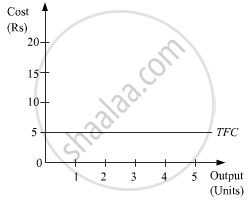# Why is the Total Fixed Cost Curve Parallel to the Horizontal Axis (X-axis)? - Economics

Short Note

Why is the Total Fixed Cost curve parallel to the horizontal axis (x-axis)?

#### Solution

Total Fixed Costs refer to those costs which are incurred by a firm in order to acquire the services of the fixed factors for production. In the short run, fixed factors cannot be varied and accordingly the fixed costs remain the same (constant) throughout all output levels. Therefore in the short run, TFC is a horizontal curve parallel to the x-axis as given in the figure.Concept: Cost - Total Fixed Cost
Is there an error in this question or solution?
2018-2019 (March) 58/4/2

Share